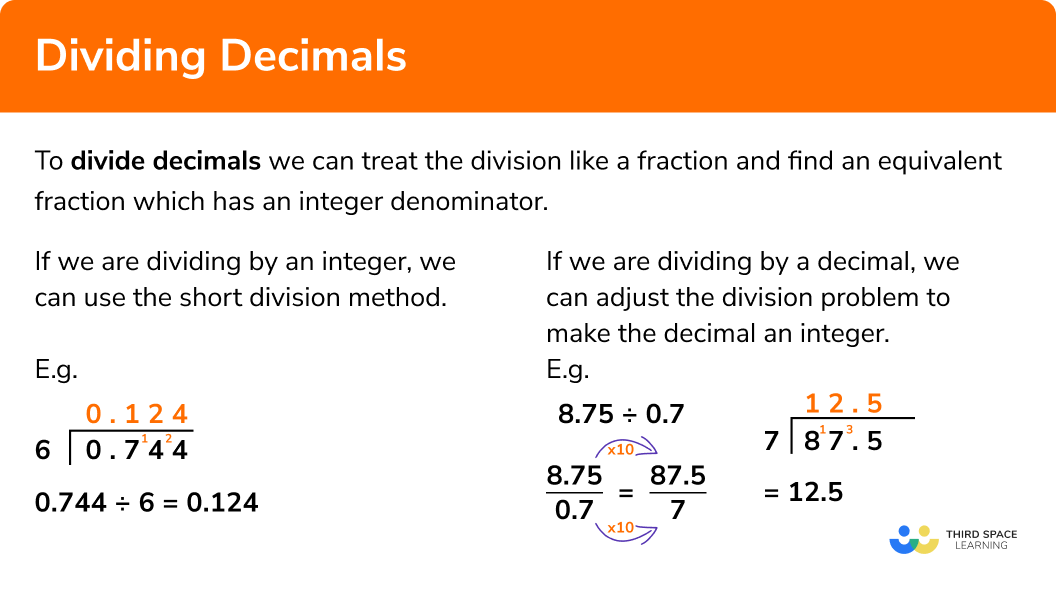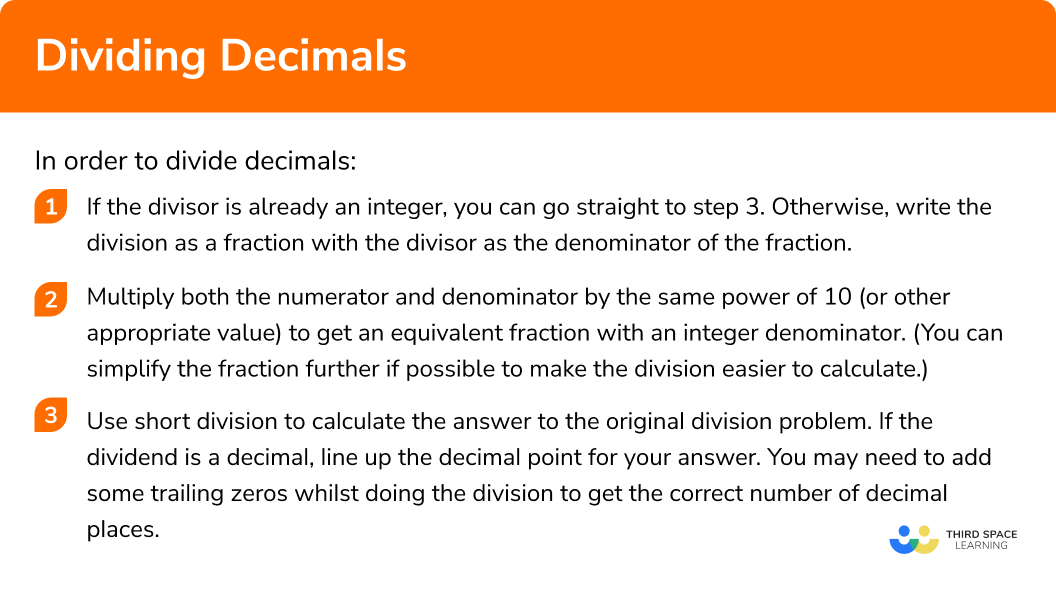# Dividing Decimals

Here we will learn about dividing decimals including how to divide decimals by a whole number, divide whole numbers by a decimal and divide decimals by decimals.

There are also dividing decimals worksheets based on Edexcel, AQA and OCR exam questions, along with further guidance on where to go next if you’re still stuck.

## What is dividing decimals?

Dividing decimals is where we divide a number into another where at least one of the numbers is a decimal.
To divide decimals we can use a variety of division methods. We will use short division for our examples but the use of long division or equivalent fractions are also appropriate methods.

Dividing decimals is important when dealing with money problems, the conversion of currency and converting between metric units. You may also encounter division problems involving areas of shapes or ratio.

If we are dividing a decimal by an integer, we can use short division method to obtain the answer.

E.g.

If we are dividing a number by a decimal, we can adjust the division problem to make the decimal an integer.

E.g.

We can treat the division like a fraction and find an equivalent fraction which has an integer denominator.
The easiest way to do this is to multiply both the numerator and denominator by the same power of ten.
This equivalent division will have the same answer as our original problem.

The answer of the division is also referred to as the quotient. The number we are dividing is known as the dividend.

### What is dividing decimals?### Recap of multiplying and dividing by 10,100,1000,…

In order to solve problems involving dividing decimals, we need to multiply and divide by powers of ten.
If we divide by a power of ten, each digit decreases in place value.

When we multiply by 10, the digits move one place to the left, so for example a digit in the hundredths column will move to the tenths column.

E.g.

If we divide by 100, the digits move two places to the rights, so for example a digit in the tens column will move to the tenths column.

E.g.

## How to divide decimals

In order to divide decimals:

1. If the divisor is already an integer, you can go straight to step 3. Otherwise, write the division as a fraction with the divisor as the denominator of the fraction.
2. Multiply both the numerator and denominator by the same power of 10 (or other appropriate value) to get an equivalent fraction with an integer denominator. (You can simplify the fraction further if possible to make the division easier to calculate).
3. Use short division to calculate the answer to the original division problem. If the dividend is a decimal, line up the decimal point for your answer. You may need to add some trailing zeros whilst doing the division to get the correct number of decimal places.

### Explain how to divide decimals in 3 steps### Related lessons on decimals

Dividing decimals is part of our series of lessons to support revision on decimals. You may find it helpful to start with the main decimals lesson for a summary of what to expect, or use the step by step guides below for further detail on individual topics. Other lessons in this series include:

## Dividing decimals examples

### Example 1: dividing a decimal by an integer

Work out:

$4.68\div6$

1. If the divisor is already an integer, you can go straight to step 3. Otherwise, write the division as a fraction with the divisor as the denominator of the fraction.

The divisor is an integer so go to step 3.

2Multiply both the numerator and denominator by the same power of 10 (or other appropriate value) to get an equivalent fraction with an integer denominator. (You can simplify the fraction further if possible to make the division easier to calculate).

Not required for this question.

3Use short division to calculate the answer to the original division problem. If the number we are dividing is a decimal, line up the decimal point for your answer and you may need to add some trailing zeros whilst doing the division to get the correct number of decimal places.

### Example 2: dividing an integer by a decimal

Work out:

$26\div0.4$

If the divisor is already an integer, you can go straight to step 3. Otherwise, write the division as a fraction with the divisor as the denominator of the fraction.

Multiply both the numerator and denominator by the same power of 10 (or other appropriate value) to get an equivalent fraction with an integer denominator. (You can simplify the fraction further if possible to make the division easier to calculate).

Use short division to calculate the answer to the original division problem. If the number we are dividing is a decimal, line up the decimal point for your answer and you may need to add some trailing zeros whilst doing the division to get the correct number of decimal places.

### Example 3: dividing a decimal by a decimal

Work out:

$32.85 \div0.9$

If the divisor is already an integer, you can go straight to step 3. Otherwise, write the division as a fraction with the divisor as the denominator of the fraction.

Multiply both the numerator and denominator by the same power of 10 (or other appropriate value) to get an equivalent fraction with an integer denominator. (You can simplify the fraction further if possible to make the division easier to calculate).

Use short division to calculate the answer to the original division problem. If the number we are dividing is a decimal, line up the decimal point for your answer and you may need to add some trailing zeros whilst doing the division to get the correct number of decimal places.

### Example 4: dividing a decimal by a decimal

Work out

$1.41\div0.15$

If the divisor is already an integer, you can go straight to step 3. Otherwise, write the division as a fraction with the divisor as the denominator of the fraction.

Multiply both the numerator and denominator by the same power of 10 (or other appropriate value) to get an equivalent fraction with an integer denominator. (You can simplify the fraction further if possible to make the division easier to calculate).

Use short division to calculate the answer to the original division problem. If the number we are dividing is a decimal, line up the decimal point for your answer and you may need to add some trailing zeros whilst doing the division to get the correct number of decimal places.

### Common misconceptions

• Reversing the multiplying steps once the division has taken place

A common error is to forget that the answer of the equivalent fraction is the same as the answer of the original division problem. If both of the numbers we are dividing have been multiplied by 10, a common mistake would be to then divide the final answer by 10 or by 100 as we need to do when multiplying decimals.

E.g.

### Practice dividing decimals questions

1. Work out 17.43\div7

2.4924924.90.249Use short division. Make sure the decimal of the quotient lines up with the decimal of the dividend.2. Work out 0.846\div3

2.820.28228228.2Use short division. Make sure the decimal of the quotient lines up with the decimal of the dividend.3. Work out 9\div0.6

1501.5150015Multiply both by 10 then use short division.4. Work out 75\div0.12

6.2562.56250.625Multiply both the numerator and denominator by 100 then use short division.5. Work out 30.04\div0.16 .

18775187.7518.7751877.5Multiply both the numerator and denominator by 100 , simplify the fraction, then use short division. Add a decimal point and trailing zeros to the dividend. Remember to line up the decimal of the quotient with the decimal of the dividend.6. Work out 87.22\div1.4 .

6.2362362.30.623Multiply both by 10 then use short division. Remember to line up the decimal of the quotient with the decimal of the dividend.### Dividing decimals GCSE questions

1.

(a) Work out 5.1\div0.17 .

(b) Work out 7.776\div12 .

(4 marks)

(a)

Method to calculate 510\div17 seen.

(1)

(1)

(b)

Attempt at short or long division.

(1)

(1)

2.

(a) The total cost of 8 identical theatre tickets is £101.20 . Find the cost of one ticket.

(b) A bag of compost holds 5kg . A single plant pot can hold 0.12kg of compost.

How many plant pots can be completely filled from one 5kg bag?

(5 marks)

(a)

A method to divide 101.2 by 8 .

(1)

(1)

(b)
A method to divide 5 by 0.12 , eg 500\div12 .

(1)

41.666 ….. seen.

(1)

(1)

3. The area of a triangle is 8.64cm^2 . The base of the triangle is 3.2cm .

Find the height of the triangle.(2 marks)

Correct start to calculation, 8.64 \times 2 =17.28 or 17.28\div 3.2 or 8.64\div1.6 or equivalent.

(1)

(1)

## Learning checklist

You have now learned how to:

• Understand and use place value for decimals, measures and integers of any size
• Use the four operations, including formal written methods, applied to integers, decimals, proper and improper fractions, and mixed numbers, all both positive and negative

## Still stuck?

Prepare your KS4 students for maths GCSEs success with Third Space Learning. Weekly online one to one GCSE maths revision lessons delivered by expert maths tutors.

Find out more about our GCSE maths tuition programme.